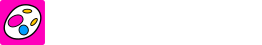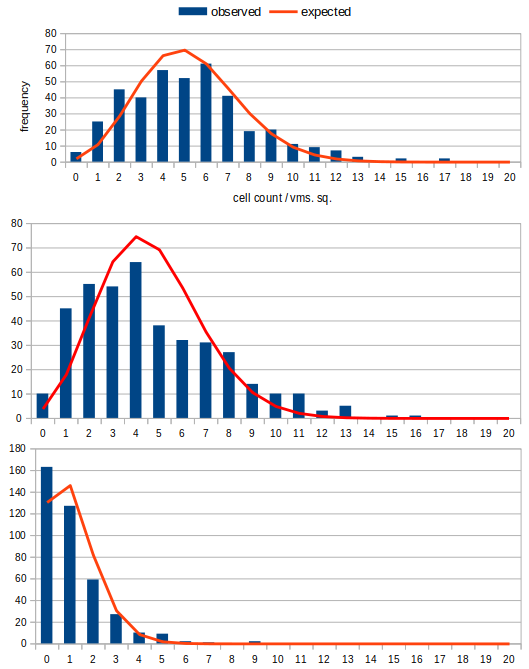### quick, easy & low-cost automated cell counting

Warning: This content is pending peer review. Please, do inquire for details.

### How Many Cells Should I Count?

When counting cells, how many is enough? 50, 100, 200? The answer rather depends on the level of accuracy and precision you want to achieve. For beer, with a desired pitch rate of 10 million cells/ml, a brewer may have experience that half a million cells (per million) either side of that mark is not consequential. The same error at 5M cells/ml might be determined unacceptable.

As with many phenomenons in nature, there is a natural, predictable distribution for the number of cells that we find in any given square of our cytometer. We can observe that our cell distributions reasonably closely follow the classic "poisson distribution". You could compare your own cell counts to the calculated Poisson distribution table, below to validate this assumption. It should be noted that this relationship is thrown off, in part, by heavily clumpy/flocculant samples. These samples can be de-flocculated by a small addition of some organic acid.

Knowing this allows us to reasonably simulate yeast counts and determine the expected accuracy and precision of our counts.

### Create a Random Cell Count

The single input to create the poisson distribution is the average we expect for any given square. If you usually count about 100 cells, give or take 10, over 5 small squares, '20' will roughly simulate your own counts. If you always diligently count the often recommended minimum of 100 cells over 5 sq's., call it '22' (ie. 22 x 5 = 110 ± 10).

### (Neubauer) Haemocytometer Chamber

The table represents a Neubauer Haemocytometer chamber. There are 25 small squares ("sm. sq.") and within each, there are 16 "very small squares" ("vsm. sq."). The number in each vsm. sq. represents the number of cells counted.

### Count Data

This chamber gives us the following counts. In this analysis we are testing the sample of small squares against the 25 squares of full chamber. If the sampling technique, particularly homogenisation - rigorous shaking of the extracted sample - has been followed, the full chamber should be sufficiently representative.

In addition to the error as a percentage, the final row shows the error as a cell count, based on a target pitch of 5 million cells per millilitre.

Total
Full Chamber Count
Full Chamber (Sm. Sq.) Avg.
Sample Count
Sample (Sm. Sq.) Average
Sample (Full Chamber) Estimate
Sample Est. Error (%)
Error @ 5M cells/ml (M. cells)

### Accuracy & Repeatability

A single count may give us a very accurate or materially inaccurate result, or indeed somewhere between. The simulation allows us to show our accuracy & repeatability over 1,000 randomised counts.

This offers a good expectation of the proportion of our real world counts that achieve each level of accuracy. By adjusting the variable and sample size of our simulated count upwards, we can show that to achieve improved repeatability, we must

(a) increase the number of cells counted in each small square, or
(b) increase the number of squares counted.

Error (±, %) Frequency Frequency (%)

### Small Squares until "True Average" (±5%) found

In statistics, somewhere near the entrance of a deep rabbit hole, we have the "Central Limit Theorum", which, amongst other things, says:

"As sample size increases, the sampling distribution more closely approximates the normal distribution, and the spread of that distribution tightens. ", statisticsbyjim.com

(For the purpose of this analysis, we're taking the full chamber to be our "population" and the sample is the number of small squares we are counting.)

In other words, the more squares we count, the closer we will get to the true average (of the distribution). Additionally, with this "distribution tightening" there are diminishing returns for our efforts.

The simulation illustrates this. We can find out how many small squares we must add to our sample before our sample average, and therefore our full chamber estimate, becomes accurate.

In the following table, we progressively add small squares until we have three consecutive mean averages which are within 5% of our "true" population average. (The counting pattern starts with the corners and centre square, then continues in that style, though randomisation makes this largely irrelevant.)

Sm. Square(s) Cumulative Count Average Error (%)

For our simulated count, the number of Small Squares required to find the true average (±5%) is -.

Again, we can consider the 1,000 iterations, where average number of Small Squares required to find the true average (±5%) is -.

And, as before, by adjusting the variable of our simulated count upwards, we can show that increasing the number of cells in each square reduces our sm. sq. sample requirement.

### Poisson Distribution

The following table allows us to compare our own real world counts to the Poisson Distribution - the assumption underlying this analysis. This table shows the probabilities and expected occurrences for any given number of cells appearing in any given "very small square". The total Expected Occurences is 16 - the number of vsm. squares in each sm. square

For a small square average count of :

Cells Probability (%) Expected OccurrencesFig. 1, Anecdotal examples of actual observations vs. the expected Poisson Distribution